NCERT Solutions for Class 9 Maths Chapter 13 Surface Areas and Volumes Ex 13.7 are part of NCERT Solutions for Class 9 Maths. Here we have given NCERT Solutions for Class 9 Maths Chapter 13 Surface Areas and Volumes Ex 13.7.

 Board CBSE Textbook NCERT Class Class 9 Subject Maths Chapter Chapter 13 Chapter Name Surface Areas and Volumes Exercise Ex 13.7 Number of Questions Solved 9 Category NCERT Solutions

## NCERT Solutions for Class 9 Maths Chapter 13 Surface Areas and Volumes Ex 13.7

Question 1.
Find the volume of the right circular cone with
(i) radius 6 cm, height 7 cm
(ii) radius 3.5 cm, height 12 cm
Solution:
(i) We have, r = 6 cm and h = 7cmQuestion 2.
Find the capacity in litres of a conical vessel with
(i) radius 7 cm, slant height 25 cm
(ii) height 12 cm, slant height 13 cm
Solution:
(i) We have, r = 7 cm and l = 25 cm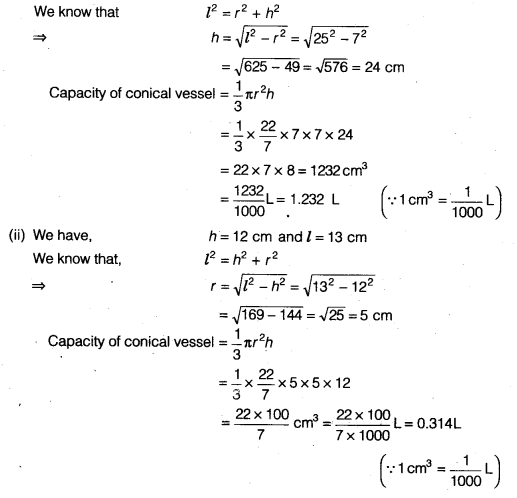Question 3.
The height of a cone is 15 cm. If its volume is 1570 cm3, find the radius of the base. (Use π = 3.14)
Solution: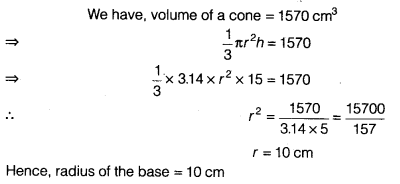Question 4.
If the volume of a right circular cone of height 9 cm is 48 cm3, find the diameter of its base.
Solution:
We have, volume of a right circular cone = 48 π cm3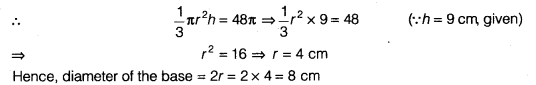Question 5.
A conical pit of top diameter 3.5 m is 12 m deep. What is its capacity in kilolitres?
Solution:
We have, diameter = 3.5 m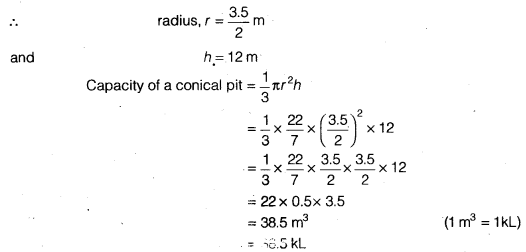Question 6.
The volume of a right circular cone is 9856 cm3. If the diameter of the base is 28 cm, find
(i) height of the cone
(ii) slant height of the cone
(iii) curved surface area of the cone
Solution:
We have, d = 28cm
⇒ r = 14 cm
∵ Volume of a right circular cone = 9856 cm3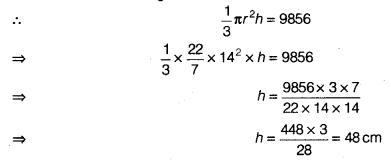Question 7.
A right triangle ABC with sides 5 cm, 12 cm and 13 cm is revolved about the side 12 cm. Find the volume of the solid so obtained.
Solution:
On revolving the right ∆ ABC about the side AB, we get a cone as shown in the adjoining figure.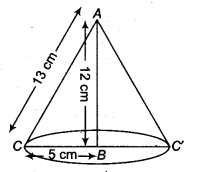∴ Volume of the solid so obtained = $$\frac { 1 }{ 3 }$$ πr2h
= $$\frac { 1 }{ 3 }$$ x π x 5 x 5 x 4 = 100π cm3
Hence, the volume of the solid so obtained is 100π cm3.

Question 8.
If the triangle ABC in the Question 7 above is revolved about the side 5 cm, then find the volume of the solid so obtained. Find also the ratio of the volumes of the two solids obtained in Questions 7 and 8.
Solution:
On revolving the right ∆ ABC about the side BC( = 5 cm),
we get a cone as shown in the adjoining figure.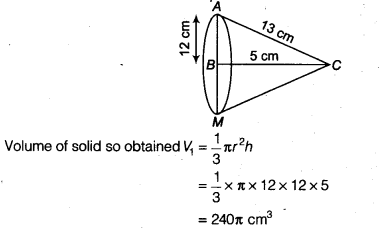In above Question 7, the volume V2 obtained by revolving the ∆ ABC about the side 12 cm i.e., V2 = 100π cm3.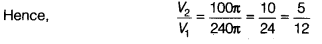Hence, the required ratio =5:12

Question 9.
A heap of wheat is in the form of a cone whose diameter is 10.5 m and height is 3 m. Find its volume. The heap is to be covered by canvas to protect it from rain. Find the area of the canvas required.
Solution: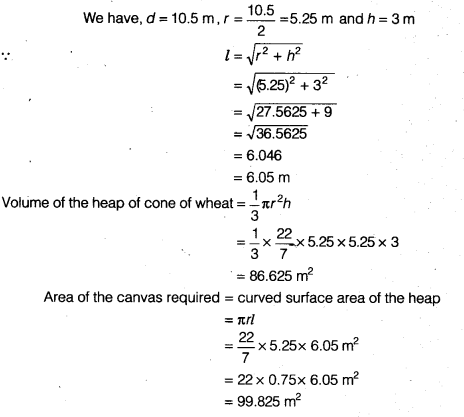We hope the NCERT Solutions for Class 9 Maths Chapter 13 Surface Areas and Volumes Ex 13.7 help you. If you have any query regarding NCERT Solutions for Class 9 Maths Chapter 13 Surface Areas and Volumes Ex 13.7, drop a comment below and we will get back to you at the earliest.